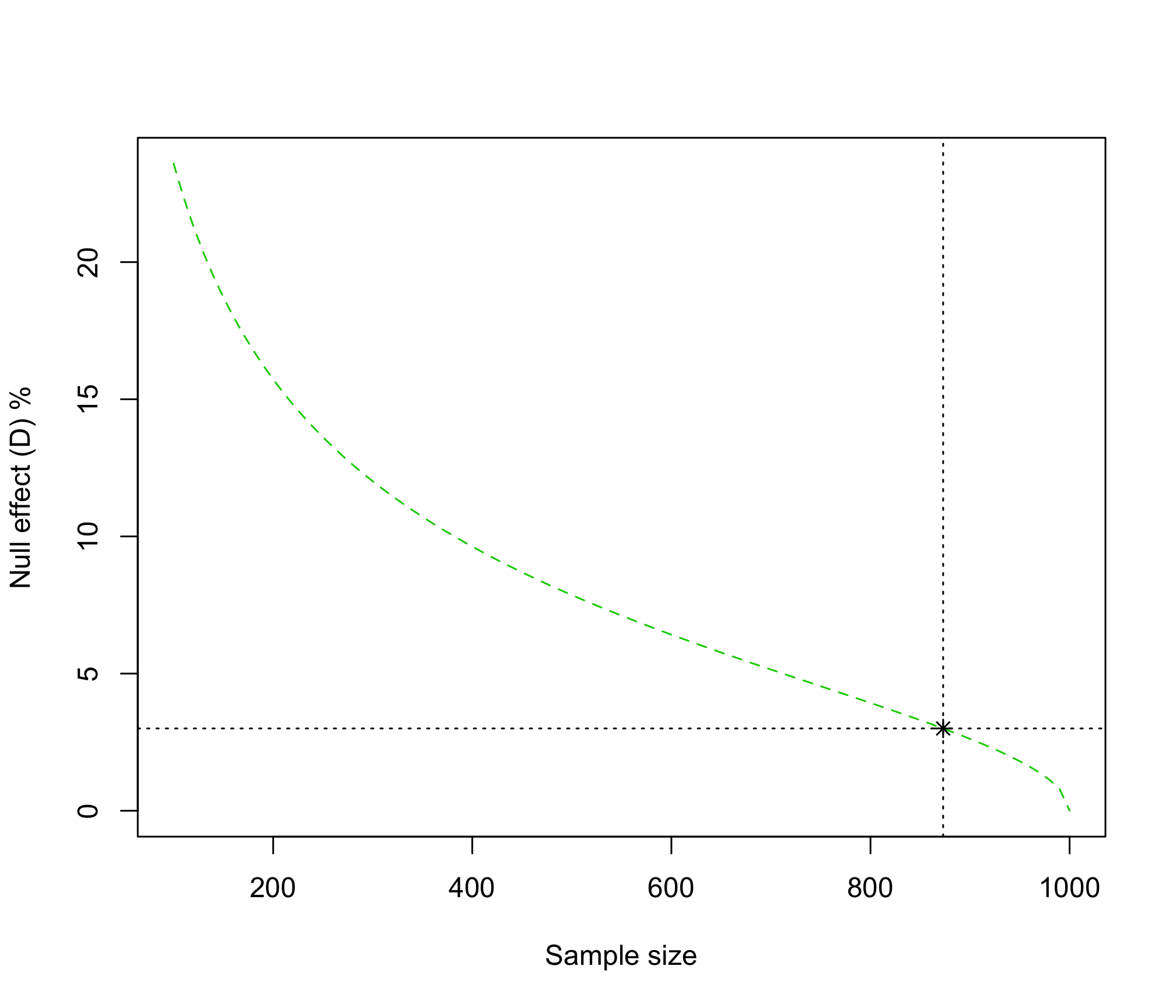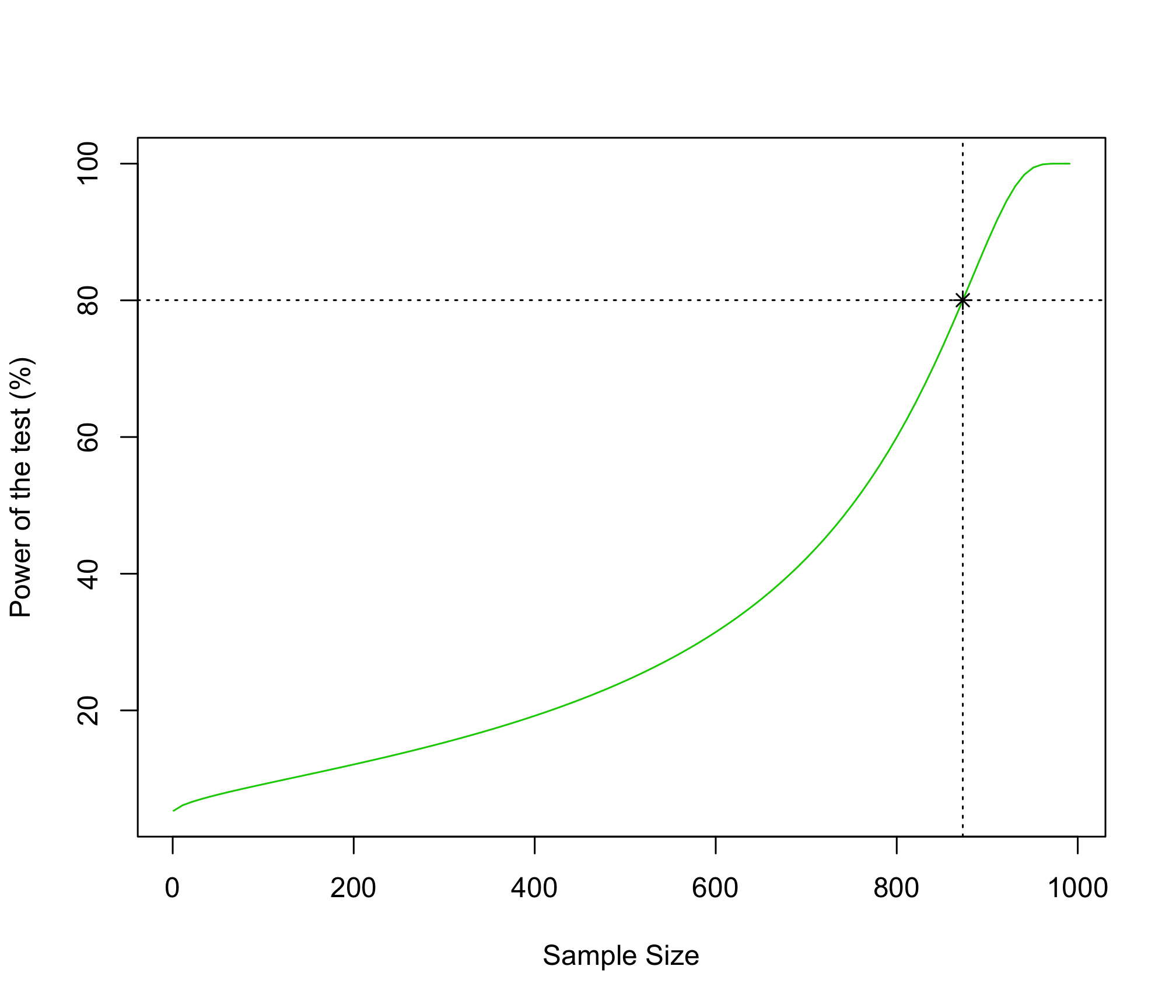Power of a Test and Sample Size in Complex Surveys

2015

There are many approaches to computing sample size. In public policy evaluation, for example, one is usually tented to check if there is statistical evidence on the impact of a intervention over a population of interest. This vignette is devoted to explain the issues that you commonly find when computing sample sizes.

The power function

Note the definition of power is related to testing an hypothesis testing process. For example, if you are interested in testing if a difference of proportions is statistically significant, then your null hypothesis may look as following:

$\begin{equation*} H_o: P_1-P_2=0 \ \ \ \ \ vs. \ \ \ \ \ H_a: P_1 -P_2 =D > 0 \end{equation*}$

Where $$D$$, known as the null effect, is any value greater than zero. You must notice that this kind of test induces the following power function, defined as the probability of rejecting the null hypothesis. Second, you note that we should estimate $$P_1$$ and $$P_2$$ by using unbiased sampling estimators (e.g. Horvitz-Thompson, Hansen-Hurwitz, Calibration estimators, etc.), say $$\hat{P}_1$$ and $$\hat{P}_2$$, respectively. Third, in general, in the complex sample set-up, we can define the variance of $$\hat{P}_1 - \hat{P}_2$$ as

$Var(\hat{P}_1 - \hat{P}_2) = \frac{DEFF}{n}\left(1-\frac{n}{N}\right)(P_1Q_1+P_2Q_2)$

Where $$DEFF$$ is defined to be the design effect that collects the inflation of variance due to complex sampling design. Usually the power function is noted as $$\beta_D$$:

\begin{align*} \beta_D &\leq Pr\left(\dfrac{\hat{P}_1-\hat{P}_2}{\sqrt{\frac{DEFF}{n}\left(1-\frac{n}{N}\right)(P_1Q_1+P_2Q_2)}} > Z_{1-\alpha} \left | \right. P_1 -P_2 =D \right)\\ &= 1-\Phi\left(Z_{1-\alpha} - \dfrac{D}{\sqrt{\frac{DEFF}{n}\left(1-\frac{n}{N}\right)(P_1Q_1+P_2Q_2)}} \right) \end{align*}

After some algebra, we find that the minimum sample size to detect a null effect $$D$$, is

\begin{align} n \geq \dfrac{DEFF(P_1Q_1+P_2Q_2)}{\dfrac{D^2}{(Z_{1-\alpha}+Z_{\beta_D})^2}+\dfrac{DEFF(P_1Q_1+P_2Q_2)}{N}} \end{align}

1. As $$D > 0$$, the sample size $$n$$ may take different values. Therefore, you should define the value of $$D$$ before drawing any sample.
2. The power function, the probability of rejecting the null hypothesis depends on $$n$$. As $$n$$ increases, $$\beta_D$$ also increases. Thereby, you should define the value of $$\beta_D$$ before drawing any sample.
3. The variance of $$\hat{P}_1 - \hat{P}_2$$ must be defined before performing the test. This way, if you know nothing about the phenomenon of interest, usually you suppose this variance to be the greatest value it can take. That occurs when $$P_1=P_2=0.5$$. This way, we have that: $Var(\hat{P}_1 - \hat{P}_2) = \frac{DEFF}{2n}\left(1-\frac{n}{N}\right)$
4. Taking 1. and 2. into account, the sample size reduces to: \begin{align} n \geq \dfrac{DEFF}{2\dfrac{D^2}{(Z_{1-\alpha}+Z_{\beta_D})^2}+\dfrac{DEFF}{N}} \end{align}
5. Recall that the assumption $$P_1=P_2=0.5$$ is not an assumption on the point estimates, but on the variance. You cannot assume point estimates. If so, why are you drawing a sample? If you already know the point estimates, why to bother in doing statistical analysis? If you know the answer, you do not carry out an expensive study!

##The ss4dpH function

The ss4dpH function may be used to plot a graphic that gives an idea of how the definition of $$D$$ affects the sample size. For example, suppose that we draw a sample according to a complex design, such that $$DEFF=2$$, for a finite population of $$N = 1000$$ units. This way, if we define the null effect to be $$D=3\%$$, then we should have to draw a sample of size $$n>$$ 873 for the probability of rejecting the null hypothesis to be 80% (default of the power), with a confidence of 95% (default of the confidence). Notice that as null effect increases, sample size decreases.

ss4dpH(N = 1000, P1 = 0.5, P2 = 0.5, D=0.03, DEFF = 2, plot=TRUE)
##  873##The b4dp function

The b4dp function may be used to plot a figure that gives an idea of how the definition of the sample size $$n$$ affects the power of the test. For example, suppose that we draw a sample according to a complex design, such that $$DEFF=2$$, for a finite population of $$N = 1000$$ units, a sample size of $$n>$$ 873, a null effect of $$D=3\%$$ and a confidence of 95%, then power of the test is $$\beta =$$ 80.0228278%. Notice that as the sample size decreases, power also decreases.

b4dp(N = 1000, n = 873, P1 = 0.5, P2 = 0.5, D=0.03, DEFF = 2, plot=TRUE)
## With the parameters of this function: N = 1000 n =  873 P1 = 0.5 P2 = 0.5 D = 0.03 DEFF =  2 conf = 0.95 .
## The estimated power of the test is  80.02283 .
##
## \$Power
##  80.02283Conclusions

You may have been fooled for some people telling you do not need a large sample size. The sample size is an issue that you have to pay a lot of attention. The conclusions of your study could have been misleaded because you draw a sample with no enough size. For example, from last figure, one may conclude that with a sample size close to 600, the power of the test is as low as 30%. That is simple unacceptable in social research.